# Calculus 3 : Normal Vectors

## Example Questions

### Example Question #41 : Normal Vectors

Determine whether the two vectors,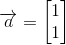and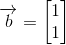, are orthogonal or not.

The two vectors are orthogonal.

The two vectors are not orthogonal.

The two vectors are not orthogonal.

Explanation:

Vectors can be said to be orthogonal, that is to say perpendicular or normal, if their dot product amounts to zero: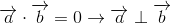To find the dot product of two vectors given the notation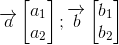Simply multiply terms across rows: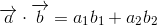For our vectors,and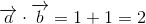The two vectors are not orthogonal. It may be observed that since these nontrivial vectors are identical, they could not be perpendicular.

### Example Question #42 : Normal Vectors

Determine whether the two vectors,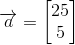and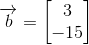, are orthogonal or not.

The two vectors are orthogonal.

The two vectors are not orthogonal.

The two vectors are orthogonal.

Explanation:

Vectors can be said to be orthogonal, that is to say perpendicular or normal, if their dot product amounts to zero:To find the dot product of two vectors given the notationSimply multiply terms across rows:For our vectors,and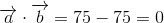The two vectors are orthogonal.

### Example Question #43 : Normal Vectors

Determine whether the two vectors,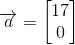and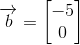, are orthogonal or not.

The two vectors are orthogonal.

The two vectors are not orthogonal.

The two vectors are not orthogonal.

Explanation:

Vectors can be said to be orthogonal, that is to say perpendicular or normal, if their dot product amounts to zero:To find the dot product of two vectors given the notationSimply multiply terms across rows:For our vectors,and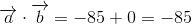The two vectors are not orthogonal.

### Example Question #44 : Normal Vectors

Determine whether the two vectors,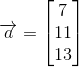and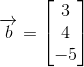, are orthogonal or not.

The two vectors are not orthogonal.

The two vectors are orthogonal.

The two vectors are orthogonal.

Explanation:

Vectors can be said to be orthogonal, that is to say perpendicular or normal, if their dot product amounts to zero:To find the dot product of two vectors given the notation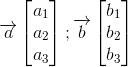Simply multiply terms across rows: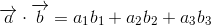For our vectors,and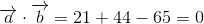The two vectors are orthogonal.

### Example Question #45 : Normal Vectors

Determine whether the two vectors,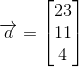and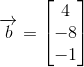, are orthogonal or not.

The two vectors are not orthogonal.

The two vectors are orthogonal.

The two vectors are orthogonal.

Explanation:

Vectors can be said to be orthogonal, that is to say perpendicular or normal, if their dot product amounts to zero:To find the dot product of two vectors given the notationSimply multiply terms across rows:For our vectors,and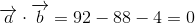The two vectors are orthogonal.

### Example Question #46 : Normal Vectors

Determine whether the two vectors,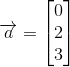and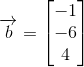, are orthogonal or not.

The two vectors are not orthogonal.

The two vectors are orthogonal.

The two vectors are orthogonal.

Explanation:

Vectors can be said to be orthogonal, that is to say perpendicular or normal, if their dot product amounts to zero:To find the dot product of two vectors given the notationSimply multiply terms across rows:For our vectors,and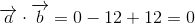The two vectors are orthogonal.

### Example Question #47 : Normal Vectors

Determine whether the two vectors,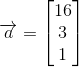and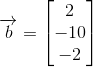, are orthogonal or not.

The two vectors are orthogonal.

The two vectors are not orthogonal.

The two vectors are orthogonal.

Explanation:

Vectors can be said to be orthogonal, that is to say perpendicular or normal, if their dot product amounts to zero:To find the dot product of two vectors given the notationSimply multiply terms across rows:For our vectors,and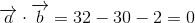The two vectors are orthogonal.

### Example Question #48 : Normal Vectors

Determine whether the two vectors,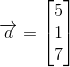and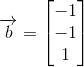, are orthogonal or not.

The two vectors are orthogonal.

The two vectors are not orthogonal.

The two vectors are not orthogonal.

Explanation:

Vectors can be said to be orthogonal, that is to say perpendicular or normal, if their dot product amounts to zero:To find the dot product of two vectors given the notationSimply multiply terms across rows:For our vectors,and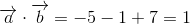The two vectors are not orthogonal.

### Example Question #49 : Normal Vectors

Determine whether the two vectors,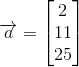and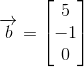, are orthogonal or not.

The two vectors are orthogonal.

The two vectors are not orthogonal.

The two vectors are not orthogonal.

Explanation:

Vectors can be said to be orthogonal, that is to say perpendicular or normal, if their dot product amounts to zero:To find the dot product of two vectors given the notationSimply multiply terms across rows:For our vectors,and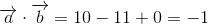The two vectors are not orthogonal.

### Example Question #50 : Normal Vectors

Determine whether the two vectors,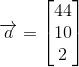and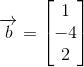, are orthogonal or not.

The two vectors are orthogonal.

The two vectors are not orthogonal.

The two vectors are not orthogonal.

Explanation:

Vectors can be said to be orthogonal, that is to say perpendicular or normal, if their dot product amounts to zero:To find the dot product of two vectors given the notationSimply multiply terms across rows:For our vectors,and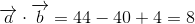The two vectors are not orthogonal.